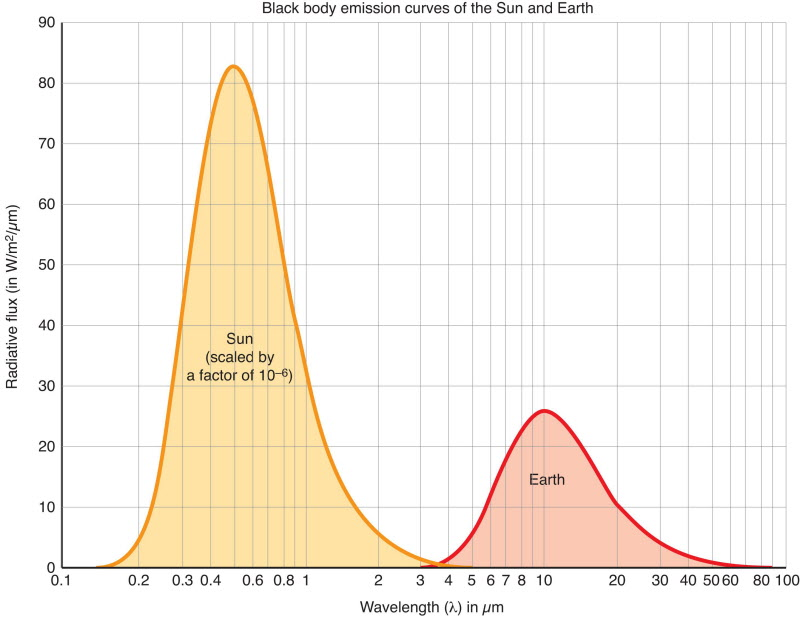An interesting (and unexpected!) application of the foundation of thermodynamics exposed earlier in this blog, is Planck's law for the black body radiation. This is the plot of the radiation of a black body, where the horizontal axis is the frequency, and the three curves correspond to three different temperatures.

The curve is similar if one replaces the frequency by the wave length. Here is a curve of sunlight with and without atmospheric absorption. Surprisingly, the one with no atmospheric absorption follows the black-body prediction quite closely!One can use the black-body model to understand at which freqencies (or wave-lengths) the earth radiates energy.## Thermodynamical Framework

Recall the modeling and notations of the foundation of thermodynamics post.

Suppose first that we found a space of microstates $$X$$ with a corresponding measure $$δx$$, an energy function $$E \colon X \to \RR$$, and an associated free energy function $A(θ) = \log \Bigl(\int \exp(θE(x)) δx\Bigr)$ with a corresponding mean energy at a given temperature given by $U(θ) = \frac{∂A}{∂θ}$

## Simple Thermodynamical System

We can immediately create a system depending on an external parameter $$V$$ by defining $E_{V}(x) := V E(x)$ From the definition of $$A$$, one obtains $A_V(θ) = A(Vθ)$ The mean energy at a given temperature is thus given by $U_V(θ) = \frac{∂A_V}{∂θ}(θ) = V \frac{∂A}{∂θ}(Vθ) = V U(Vθ)$

## Family of Systems

We postulate that we have a family of such systems, that is, for any value of the extensive variable $$V$$, there are $$g(V) \dd V$$ such systems. Because of the dimension, the density of state $$g$$ turns out to have to be a quadratic polynomial: $g(V) = V^2$ We can already find out how the total energy depends on the temperature! Indeed, we just compute $E(θ) = \int_{0}^{∞} V^2 U_V(θ) \dd V = \int_{0}^{∞} V^3 U(Vθ) \dd V = θ^4 C$ where $$C$$ is a constant, namely the integral $$C = ∫_{0}^{∞} V^3 U(V) \dd V$$.

What we have found is the Stefan–Boltzmann law:

$E(θ) \sim θ^4$

## Microstate Space

It remains to find an interesting example of microstate space $$X$$ and its associated measure $$δx$$.

It turns out that one such example is as follows. Choose $X = \NN$ With its associated counting measure. Choose now the energy function $E(n) = n$ This gives $A(θ) = \log\Bigl(\sum_{k=0}^{∞} \exp(kθ)\Bigr) = -\log(1-\exp(θ))$ since the sum is a geometric sum. This is only defined for $θ < 0$ From this, we obtain $U(θ) = \frac{\exp(θ)}{1-\exp(θ)} = \frac{1}{\exp(-θ) -1}$ so we finally obtain $U_V(θ) = \frac{V}{\exp(-Vθ) -1}$

The total energy $$E(θ)$$ at a given temperature $$θ$$ becomes $E(θ) = \int \frac{V^3}{\exp(-Vθ)-1} \dd V$ The term inside the integral is Planck's law! In other words, we have $E(θ) = \int B(V,θ) \dd V$ with

$B(V,θ) = \frac{V^3}{\exp(-Vθ)-1}$

This is the function which is plotted in the beginning of this post for various values of $$θ$$.